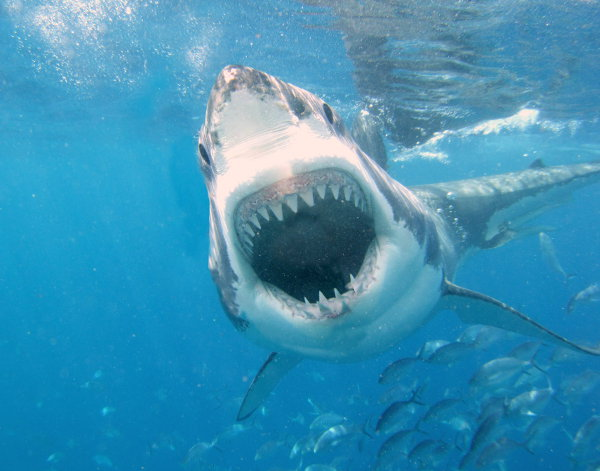# Scientific Notation

Scientific Notation (also called Standard Form) is used by scientists to measure very large objects such as the size of the sun and very small objects such as cells.

In scientific notation, there is only one digit before the decimal point.

The number of places that the decimal point has moved is the index of the 10.

We do not write the zeros before or after the actual digits unless the zeros are within the digits.

## Example One - Diameter of Jupiter

The diameter of Jupiter, the largest planet in our Solar System, is 140 000 kilometres.
Write this number in scientific notation.

140 000 = 1.4×105

## Example Two - Distance to the Sun

The distance to the Sun, our closest star, is 149 600 000 kilometres.
Write this number in scientific notation.

149 600 000 = 1.496×108

## Example Three - Size of an Object Seen with the Naked EyeThe thickness of a butterfly wing is 0.00035 centimetres.
Write this number in scientific notation.

0.00035 = 3.5×10 –4
(Notice that the index is negative to indicate that the decimal point was moved in the other direction.)

## Example Four - Size of a CellThe thickness of a cell is 0.0000608 centimetres.
Write this number in scientific notation.

0.000608 = 6.08×10 –5
(Notice that the zero within the digits is included.)

## Did You Know That...?

Nanotechnology is a fascinating new scientific field. A nanometre is one billionth of a metre (1/1000 000 000 of a metre). Want to find out more? Great! Research these terms: nanotechnology, nanotube, Bucky balls.

## Example Five - Probability of Being Attacked by a SharkAccording to the Florida Museum of Natural History, the number of unprovoked shark attacks worldwide in 2010 was 79 attacks. What is my chance of being attacked by a shark if I go swimming? Write the answer in scientific notation.

Number of people in the world = 7 billion = 7 000 000 000
Probability (Attacked by shark) = 79 ÷ 7 000 000 000 = 0.000011 = 1.1×10 –5

## Example Six - Multiplying Numbers in Scientific Notation

2.3×104  ×  4.5×105  ×  3.8×10 –3

(2.3 × 4.5 × 3.8) × (104 × 105 × 10 –3)
= 39.33×106
= 3.933×107

## Questions

Convert these to scientific notation.
Q1. 389 000
Q2. 0.00508

Convert these to normal decimal numbers.
Q3. 5.2×108
Q4. 3.42×10 –2

Calculate and then leave your answer in scientific notation.
Q5. 3.5×10 –2  ×  8.9×106
Q6. 8.3×10 –4  ×  2.5×10 –3